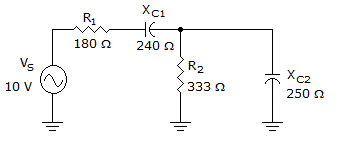# Electronics - RC Circuits - Discussion

Discussion Forum : RC Circuits - General Questions (Q.No. 3)
3.What is the voltage drop across R1 in the given circuit?

10 V
4.80 V
4.00 V
5.80 V
Explanation:
No answer description is available. Let's discuss.
Discussion:
20 comments Page 1 of 2.

Tabasum parween said:   2 years ago
(1)

Grandaphi said:   4 years ago
Doesn't (1/333-1/250i)^-1 = 120.03 - 159.88i not 120 + 159.88i?

And I get 3.6V.

Samar said:   5 years ago
Here;

1. Get the inverse (1/333 - 1/250i)^-1 to get the impedance since it is in admittance form, then it will be equal to 120.03 + 159.88i.
2. Then next step is to add that to (180 - 240i).
3. And, (120.03 + 159.88i) + (180 - 240i) = 300.09 - 80.12i.
4. Then convert that to Polar form which is equal to 310.55 <-14.95.
5. Now the total impedance is 310.55, and by voltage divider formula Vr1 = (10/310.55)(180) = 5.8 volts.
(1)

Safna said:   6 years ago

Rose said:   7 years ago
I want an answer to my question.

Suppose we have a circuit where the ratio of the complex amplitude of the particular integral to the amplitude of the driving sinusoid is

VpVi = jωRC1 + jωRC.

Given that R = 0.82kΩ and C = 10.0nF we will compute the magnitude and phase of this ratio for various frequencies.

For frequency f = 170Hz what is the magnitude of VpVi?

Divyanshu Shekhar said:   7 years ago
I think here XL1 = 240 ohm not XC 1=240 ohm.

When XL 1 = 240 ohm, then calculate:

z1 = 180 + j240
z2 = 333 * -j250/(333-j250)
= 120.03 -j159.88
= 120-j160 (approx.)
z = z1 + z2
= 300 + j80

z = sqrt[(300)2 + (80)2]
=310.48 ohm = 310.5 ohm(approx.).

i = v/z = 10/310.5 = 0.0322 A.
Voltage drop across r1 = 180 * 0.0322.
= 5.79 volts.
= 5.8 volt(approx).

Also, phase angle, tan(θ) = 80/300 = 0.26666 = 0.267(approx).
So, θ = Arctan(0.267).
= 14.95 degree.

PrivUsr said:   8 years ago

Which one is correct? Anybody tell me.

Abhiee said:   8 years ago
Let me tell in more simpler way.

R1 and XC1 are series to source. So z1 = sqrt (R1^2+XC1^2).

R2 and XC2 are parallel to source. So z2 = 1/sqrt ((1/R2)^2+(1/XC2)^2).

Then z = z1+z2. Voltage drop = R1/z*vs.

Alex said:   8 years ago
No. Just simply, no.

You guys are all doing this wrong and the answers are wrong.

You can't simply combine the parallel R2||Xc2 in the traditional method. You have to remember that capacitive reactance is at an angle of -90°.

When you combine that parallel branch, you have to convert back and forth between polar and rectangular forms as appropriate (addition in rectangular, multiplication/division in polar).

So let me walk you through this:

Req=R2||X2.
Req=333||-j250.

Req=1/((1/333)+(1/250<-90°)) <--in polar for division.
Req=1/(0.003+j 0.004) <--in rectangular for addition.

Req=1/(0.005<53°) <--Convert to a vector/rectangular.
Req=200<-53° <-- That is the equivalent resistance.

Now, convert that back to rectangular and add R1 and Xc1:

200<-53° = 120-j159.73.

Zt = (120-j159.73)+(180-j240).

Zt = 300-j400 <-- (rounded to -j400).

Zt = 500<-53° <-- (polar representation).

Now:

It = Vt/Zt=10V/500<-53°) = 0.02 A < 53°.

VR1 = It*R1 = 0.02 A*180Î© = 3.6 V.

Sangamesh said:   9 years ago
Since the parallel combination of xc2 and R2 = 142.79.

Therefore z = square root(180^2+240^2+142.79^2) = 332.25.

Hence Vr1 = 10*180/332.25 = 5.8v.